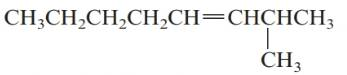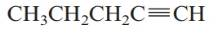Chapter 20.7, Problem 20.4SC### Introductory Chemistry: A Foundati...

9th Edition
Steven S. Zumdahl + 1 other
ISBN: 9781337399425

#### Solutions

Chapter
Section### Introductory Chemistry: A Foundati...

9th Edition
Steven S. Zumdahl + 1 other
ISBN: 9781337399425
Textbook Problem
1 views

# Exercise 20.4 Name the following molecules. a.b.Interpretation Introduction

(a)

Interpretation:

The name of the given molecule should be determined.

Concept Introduction:

Compounds consist of carbon and hydrogen is known as hydrocarbons. Unsaturated hydrocarbon having double bond is known as alkene having general molecular formula CnH2n. Unsaturated hydrocarbon having triple bond is known as alkyne having general formula CnH2n1

Rules of naming alkenes are:

1. First choose the longest continuous chain of carbon atoms having double bond known as parent chain.
2. The numbering of parent chain should be done in a way that the double bond and substituents get the lowest number.
3. The root name of the carbon chain is same as in case of alkanes, but “−ane” ending is replaced by “−ene”.
4. The appropriate name should be given to every alkyl group and denote its position on the parent chain with the number.
5. The alkyl groups are written in alphabetical order.
Explanation

The given structure is

1. The longest continuous chain contains eight carbon atoms and the prefix “oct” is used...

Interpretation Introduction

(b)

Interpretation:

The name of the given molecule should be determined.

Concept Introduction:

Compounds consist of carbon and hydrogen is known as hydrocarbons. Unsaturated hydrocarbon having double bond is known as alkene having general molecular formula CnH2n. Unsaturated hydrocarbon having triple bond is known as alkyne having general formula CnH2n1

Rules of naming alkynes are:

1. First choose the longest continuous chain of carbon atoms having triple bond known as parent chain.
2. The numbering of parent chain should be done in a way that the triple bond and substituents get the lowest number.
3. The root name of the carbon chain is same as in case of alkanes, but “−ane” ending is replaced by “−yne”.
4. The appropriate name should be given to every alkyl group and denote its position on the parent chain with the number.
5. The alkyl groups are written in alphabetical order.

### Still sussing out bartleby?

Check out a sample textbook solution.

See a sample solution

#### The Solution to Your Study Problems

Bartleby provides explanations to thousands of textbook problems written by our experts, many with advanced degrees!

Get Started

#### Find more solutions based on key concepts